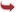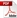International Journal of Scientific and Research Publications

#### IJSRP, Volume 6, Issue 10, October 2016 Edition [ISSN 2250-3153]FIND OUT nth ORDER ROOT OF A NUMBER BY DIVISION METHOD (USING THIS THEOREM CALCULATION 3rd, 4th, 5th ROOT OF ANY REAL NUMBER & DEDUCE (1+f(x))1/n expansion where f(x) is an algebraic function)
Krishna Kumar Kharwar
Abstract: Find out nth order root of any real number by division method
[VIEW FULL PAPER][DOWNLOAD]

Reference this Research Paper (copy & paste below code):

Krishna Kumar Kharwar (2018); FIND OUT nth ORDER ROOT OF A NUMBER BY DIVISION METHOD (USING THIS THEOREM CALCULATION 3rd, 4th, 5th ROOT OF ANY REAL NUMBER & DEDUCE (1+f(x))1/n expansion where f(x) is an algebraic function); Int J Sci Res Publ 6(10) (ISSN: 2250-3153). http://www.ijsrp.org/research-paper-1016.php?rp=P585937